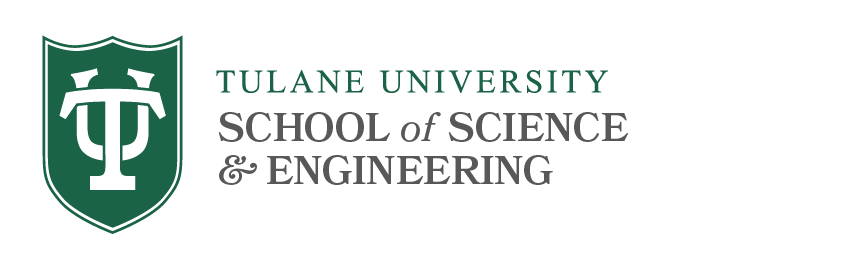# Probability & Statistics Qualifying Exams

## Syllabus Topics

The following topics & references will prepare you for the exam.

### Mathematical Statistics

Topics for Mathematical Statistics:

• The Method of Moments: basic properties and asymptotics.
• Exponential families: canonical and curved. Canonical exponential families: natural parameter space, identifiability, convexity. Location and scale families.
• Sufficient, minimal, ancillary, complete statistics. Factorization theorem, conditions for minimality or completeness. Sufficiency theory for canonical exponential families. Basu's theorem.
• Bayesian theory: prior and posterior distributions, loss functions, Bayes risk, Bayesian point estimation and hypothesis testing.
• UMVU estimation: risk and loss functions, Information Inequalities. Fisher information, uniqueness of the UMVU estimator. Rao-Blackwellization of estimators. Risk-function-based optimality and sufficiency. Information inequalities for exponential families.
• Hypothesis Testing: simple and composite hypotheses, randomized and non-randomized tests, size, power, p-values. The Neyman-Pearson Lemma. Optimality of tests: UMP and UMPU tests. Generalized Likelihood Ratio tests. Asymptotics of likelihood ratio tests.
• Asymptotics: Convergence in law and in probability, the Delta Method and Taylor approximations. Consistent roots of the likelihood equation. Maximum likelihood estimation and asymptotic efficiency, asymptotic theory for Bayesian estimators.

References:
1. Lehmann, E., and G. Casella (1998), Theory of Point Estimation, Springer, 2nd edition.
2. Lehmann, E., and Romano, J. (2005), Testing Statistical Hypotheses, Springer, 3rd edition.
3. Bickel, P. and Doksum, K. (2002), Mathematical Statistics: Basic Ideas and Selected
Topics, Vol. I, 2nd edition, Pearson Prentice Hall.
4. Ferguson, T. (1967), Mathematical Statistics: a Decision Theoretic Approach, Academic Press.
5. Berger, J. (2013), Statistical Decision Theory and Bayesian Analysis, Springer Science &
6. Casella and Berger (2002), Statistical Inference, 2nd edition, Duxbury.

### Probability

Topics for Probability:

• Probabilistic models for an experiment: sample spaces, algebras, sigma-algebras, Borel sets. Kolmogorov's axioms and the construction of measures (Caratheodory extension).  The Lebesgue measure and probability measures.  Conditional probability, the law of total probability, independence.  Sequences of events, liminf and limsup.  The monotone class lemma.
• The equivalence between random variables and distribution functions.  The characterization of distribution functions and the Lebesgue decomposition.  Random vectors and their distribution functions.  The multivariate normal distribution.  Distribution functions of random vectors, independence.  The distribution of functions of random vectors and the Jacobian method.  The Borel-Cantelli lemma and the Borel 0-1 law.  Tail sigma-algebras and Kolmogorov's 0-1 law.
• The Lebesgue-Stieltjes integral.  Expectations of functions of random variables and vector, changes of variables under the Lebesgue-Stieltjes integral.  Jensen's inequality, Markov's inequality.  Moments and covariance.  The monotone convergence and the dominated convergence theorems.
• Convergence of random variables: in probability, almost sure and in Lp.  The method of truncation of random variables.  The general weak law of large numbers, Chebyshev's weak law, Khinchin's weak law.  Kolmogorov's 1-st strong law, Kolmogorov's strong law of large numbers and its converse.
• Characteristic functions and their properties.  The inversion formula.  Convergence in distribution: Helly-Bray's theorem, Paul Levy's continuity theorem.  Characteristic functions of random vectors, the Cramer-Wold device.  Scheffe's theorem.  The convergence of continuous functions of random variables, Slutsky's theorem.  The Delta method.  The Bochner-Khintchin theorem.
• The Lindeberg-Feller and Lyapunov central limit theorems.  The "converse" to the Lindeberg-Feller theorem.  The central limit theorem in the multivariate setting.
• Conditional distributions and expectations in terms of Lebesgue-Stieltjes integrals.  The conditional distribution of X given Y: conditions for existence.  Well-behaved cases: Y is discrete, X and Y are independent, X and Y have a joint density.  Regular conditional distributions.  Conditional expectations: existence and properties, the law of iterated expectations.

References:
1. Resnick, S. (1998), A Probability Path, Boston, Birkhäuser.
2. Shiryaev, A.N. (2000), Probability Theory, New York, Springer-Verlag.
3. Ash, R. (2008), Basic Probability Theory, New York, Wiley.
4. Jacod, J. and Protter, P. (2004). Probability Essentials, 2nd edition, Springer.

### Linear Models (for MS students only)

Topics for Linear Models:

• Multiple and Simple Least Squares Linear Regression developed from Linear Algebra, with emphasis on the geometric interpretation and Perpendicular Projection Matrices, orthogonal matrices, t and F statistics from this viewpoint.
• Gauss Markov Theorm
• Sampling Distributions and Statistics Inference for Regression Parameters
• F tests for nested models and the general linear hypothesis
• Weighted Linear Regression
• One-way and Two-way ANOVA
• Identifiability and Estimability of Parameters
• Regression Diagnostics--residuals, plot and heteroscedasticity, leverage, outliers, influential points, Cook's distance
• Model Section and Multicollinearity--R squared, Mallows Cp, AIC, BIC, forward and backward and stepwise selection

References:
1. Christensen, Plane answers to Complex Questions
2. Weisberg, Applied Linear Regression